# 高强中模复合材料开孔层压板边缘应力分析研究Edge Stress Analysis of High Strength Medium Modulus Composite Laminates with Openings

DOI: 10.12677/MS.2021.112016, PDF, HTML, XML, 下载: 39  浏览: 67

Abstract: In order to solve the engineering problem of large area delamination at the edge of T800 carbon fiber reinforced composite laminate under low stress level during tensile fatigue test, the influence of width diameter ratio (the ratio of width to diameter) on the edge stress concentration was ana-lyzed. The stress distribution of five kinds of specimens with aspect ratio of 6~10 under tensile load is simulated by numerical simulation. The results show that the edge effect always exists and will not disappear with the increase of aspect ratio, but will weaken with the increase of aspect ratio.

1. 引言

2. 数值仿真模型与方法

2.1. 数值模型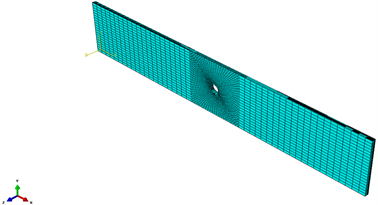Figure 1. Numerical model of open hole tensile specimen

2.2. 数值模型边界条件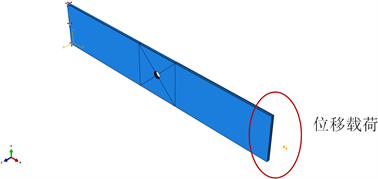Figure 2. Boundary conditions

2.3. 材料力学性能参数Table 1. Basic mechanical property parameters of T800 carbon fiber reinforced composites

3. 数值仿真模型分析

3.1. 数值模型有效性验证

3.2. 宽径比对开孔层压板边缘应力影响分析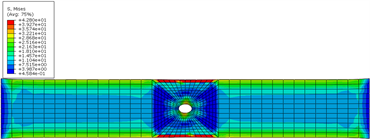(a) 界面Mises应力云图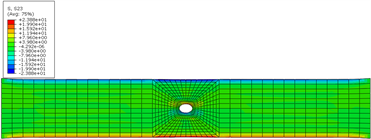(b) 界面2~3方向剪切应力云图

Figure 3. Stress nephogram of interlaminar interface of laminate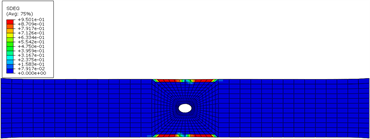(a) 界面1损伤云图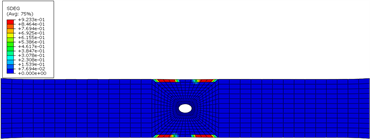(b) 界面2损伤云图(c) 界面3层间损伤云图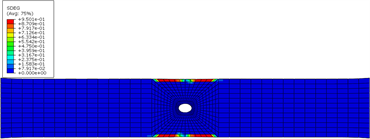(d) 界面4层间损伤云图

Figure 4. Typical interlaminar damage nephogram of laminate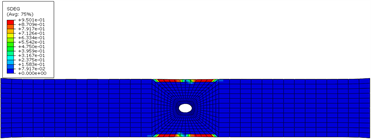(a) 界面1损伤云图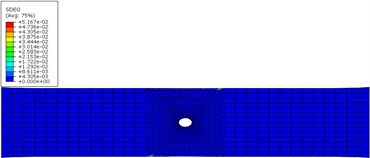(b) 界面2损伤云图

Figure 5. Typical interface damage of open hole tensile specimen (W/D = 7) at 350 MPa stress level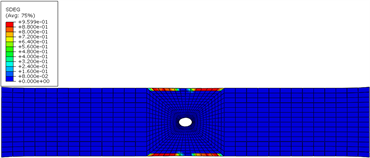(a) 界面1损伤云图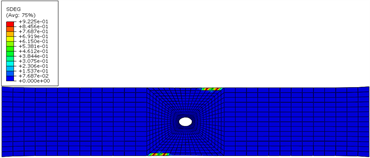(b) 界面2损伤云图

Figure 6. Typical interface damage of open hole tensile specimen (W/D = 7) at 390 MPa stress level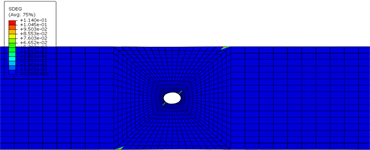(a) 界面1损伤云图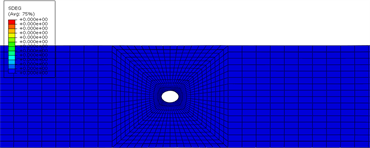(b) 界面2损伤云图

Figure 7. Typical interface damage of open hole tensile specimen (W/D = 8) at 350 MPa stress level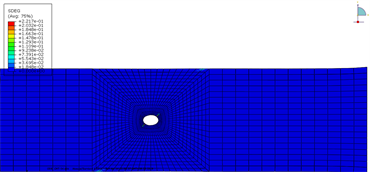(a) 界面1损伤云图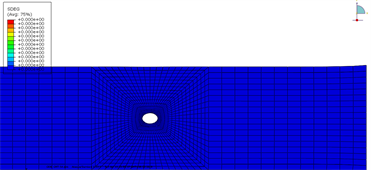(b) 界面2损伤云图

Figure 8. Typical interface damage of open hole tensile specimen (W/D = 9) at 350 MPa stress level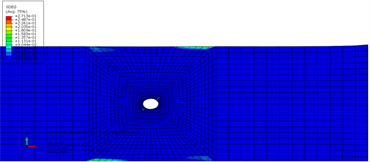(a) 界面1损伤云图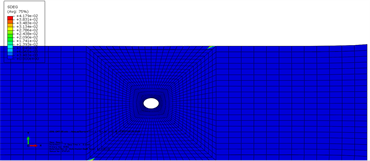(b) 界面2损伤云图

Figure 9. Typical interface damage of open hole tensile specimen (W/D = 10) at 350 MPa stress level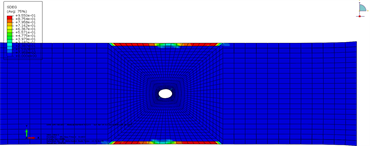(a) 界面1损伤云图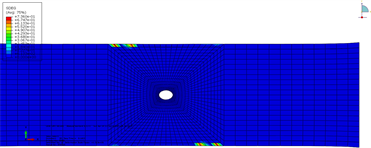(b) 界面2损伤云图

Figure 10. Typical interface damage of open hole tensile specimen (W/D = 10) at 412 MPa stress level

4. 结论

  Salamon, N.J. (1978) Interlaminar Stresses in Composite Laminatein Bending. Fibre Science and Technology, 11, 305-317. https://doi.org/10.1016/0015-0568(78)90020-9  Altus, E., Rotem, A. and Shmueli, M. (1980) Free-Edge Effect in Angle-Ply Laminates—A New Three-Dimensional Finite Difference Solution. Journal of Composite Materials, 14, 21-30.  Pipes, R.B. and Pagano, N.J. (1970) Interlaminar Stresses in Composite Laminates under Axial Extension. Journal of Composite Materials, 4, 538-548. https://doi.org/10.1177/002199837000400409  Bhaskar, K., Varadan, T.K. and Jacob, C. (2000) Free-Edge Stresses in Laminated Cylindrical Shells Due to Axisymmetric Transverse Loads. Aeronautical Society of India, 52, 26-38.  Wang, A.S.D. and Crossman, F.W. (1977) Some New Results on Edge Effect in Symmetric Composite Laminates. Journal of Composite Materials, 11, 92-106. https://doi.org/10.1177/002199837701100110  Tang, S. (1975) A Boundary Layer Theory-Part I: Laminated Composites in Plane Stress. Journal of Composite Materials, 9, 33-41. https://doi.org/10.1177/002199837500900104  Tang, S. and Levy, A. (1975) A Boundary Layer Theory-Part II: Extension of Laminated Finite Strip. Journal of Composite Materials, 9, 42-45. https://doi.org/10.1177/002199837500900105